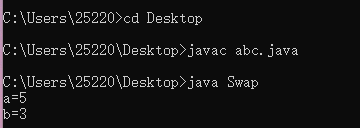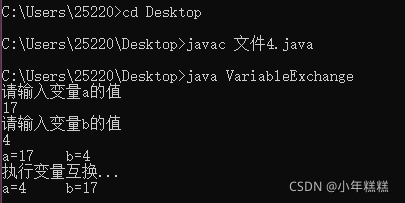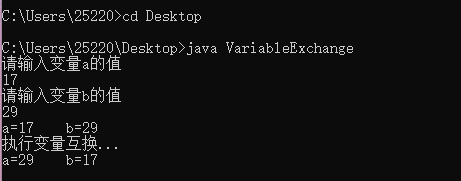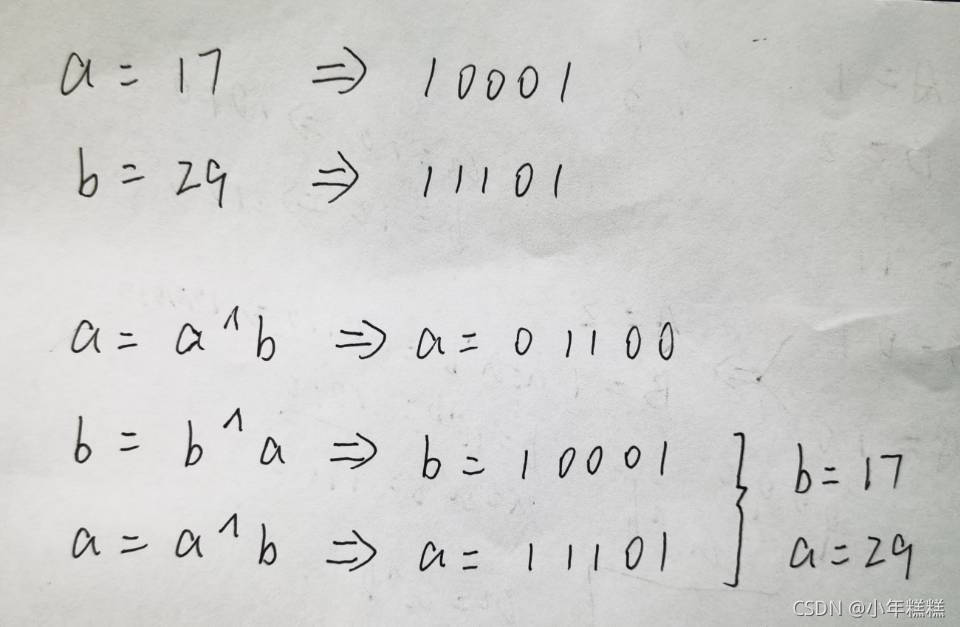﻿ Java中不用第三个变量来互换两个变量的值 - 自由资讯

# Java中不用第三个变量来互换两个变量的值

```class Swap{
public static void main(String[] args){
int a = 3;
int b = 5;

int c;
c = a; //把a的值先存到c
a = b; //把b存给a
b = c; //把存在c的值倒赋给b

System.out.println("a="+a); //a==5
System.out.println("b="+b); //b==3
}
}
```（1）运用加法的性质来实现两个数的互换

```import java.util.Scanner;
class VariableExchange{
public static void main(String[] args){
Scanner scan = new Scanner(System.in);          //创建扫描器
System.out.println("请输入变量a的值");
long a = scan.nextLong();                       //接收第1个变量值
System.out.println("请输入变量b的值");
long b = scan.nextLong();                       //接收第2个变量值
System.out.println("a=" + a + "\tb=" + b );
System.out.println("执行变量互换...");
a = a + b;
b = a - b;
a = a - b;
System.out.println("a=" + a + "\tb=" + b );
}
}
```（2）运用异或运算实现两个数的互换（硬菜）

```import java.util.Scanner;
class VariableExchange{
public static void main(String[] args){
Scanner scan = new Scanner(System.in);          //创建扫描器
System.out.println("请输入变量a的值");
long a = scan.nextLong();                       //接收第1个变量值
System.out.println("请输入变量b的值");
long b = scan.nextLong();                       //接收第2个变量值
System.out.println("a=" + a + "\tb=" + b );
System.out.println("执行变量互换...");
a = a ^ b;                                      //执行变量互换
b = b ^ a;                                      //执行变量互换
a = a ^ b;                                      //执行变量互换
System.out.println("a=" + a + "\tb=" + b );
}
}
```﻿